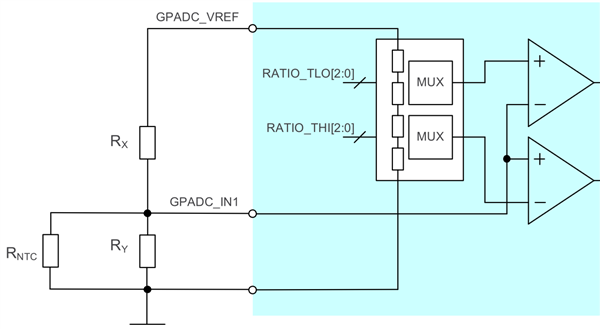If you have a related question, please click the "Ask a related question" button in the top right corner. The newly created question will be automatically linked to this question.

# TPS80032/about Temperature Monitoring with External NTC Resistor

Other Parts Discussed in Thread: TPS80032

Hi,

Could you tell me about TPS80032 NTC thermistor monitoring?
Is there the hysteresis for this detection?
It is awkward for the customer that an IC repeats charge start and stop.Best Regards,
Yusuke/Japan Disty

• Hey Yusuke,

The NTC thermistor monitoring uses the GPADC_IN1 and compares the voltage there to the RATIO_TLO and RATIO_THI defined in the OTP document for a particular OTP multiplied by the GPADC_VREF. We recommend the Rx and Ry to linearize the NTC response.

The values of Rx and Ry can be calculated with a known R(T=25) and beta value for the thermistor combined with the TH (high temperature) and TL (low temperature):
Thermistor resistance at TH: RT_Cold = RT_25 x e[B x [1/(273.15 +TC) - 1/(273.15 +T_25)]
Rx = (1-Ratio_THI) / (Ratio_THI x RT_Cold x Ry) / (RT_Cold + Ry)
Thermistor resistance at TL: RT_Hot = RT_25 x e[B x [1/(273.15 +TH) - 1/(273.15 +T_25)]
Ry = RT_Cold x RT_Hot x (k-1) / [RT_Cold - (k x RT_Hot)]
where k = (Ratio_THI/Ratio_TLO) x (1-Ratio_TLO)/ (1-Ratio_THI)

There is no built-in hysteresis for the GPADC, so charging will be re-enabled as soon as the voltage it senses returns to a value between VREF*RATIO_TLO and VREF*RATIO_THI.

Thanks,
Kevin LaRosa
• Kevin-san,

Thank you for your kind support.
I tell a setting method of Rxy to a customer.

Let me ask you one more thing.
At the time of over temp(bat temp), what kind of status are GPADC_VREF and GPADC_IN1?

Best Regards,
Yusuke/Japan Disty

• Yusuke-san,

I don't understand the question.

The GPADC_VREF will be on as long as the GPADC is enabled. The GPADC_IN1 input at an over temp condition should be below RATIO_THI * GPADC_VREF.

Thanks,
Kevin LaRosa
• Kevin-san,

Thank you for your information and support.
The customer is troubled with the following phenomena.

◇Condition
・Setting RATIO_HI the (High Temperature) =45 ℃
・Ambient temperature =40℃
◇Phenomenon
・Battery charge Start (0.5sec)⇒Battery chage Stop (4sec)⇒Battery charge Start (0.5sec)⇒...This loop.

Is this phenomenon right by understanding to repeat charge anda stop by Battery temperature monitoring?
Could you tell me if you have any information.

Best Regards,
Yusuke/Japan Disty

• Hi Yusuke-san,

There is no hysteresis on the battery charging as a function of temperature, so if the voltage on the GPADC_IN1 is near VREF*RATIO_TLO, then there is potential for this to occur. Have they taken scope shots of the trace near the ball? If there is noise on the GPADC_VREF, GPADC_IN0, REFGND, or GND_ANA then it could explain why they see triggering early. Alternatively, if the NTC is non-ideal then there is the same potential of early triggering.

Thanks,
Kevin LaRosa